# Positive and Negative Integer Exponents

An integer is a number with no fractional part that includes the counting numbers {1, 2, 3, 4, â¦} , zero {0} and the negative of the counting numbers {- 2, -1, 0, 1, 2}. An exponent of a number says how many times to use that number in a multiplication.
Let's start by reviewing the rules for exponents

I. Multiplying

When you multiply same bases you add exponents.

x4 •x5 = x4+5 = x9

What if an exponent is negative? Same thing add exponents.

x6 •x-4 = x6+(-4) = x2

What if there is more than one variable? Do each base separately.

(xy6)(x3y4) = x1+3 y6+4 = x4 y10

What if there is a coefficient in front of the variable?

3x2 •-2x3 =

(3 •-2)•(x2 • x3) =   Use the commutative property to rearrange
-6x5   multiply the coefficients and add exponents

II. Dividing

When you divide same bases you subtract exponentsWhat if there is more than one variable? Do each base separately.What if there is a coefficient in front of the variable? Divide the coefficients.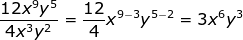What if the exponent is negative?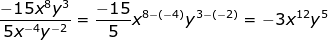III. Raising a power to a power

When you raise a power to a power you multiply exponents.

(x3)5 = x3•5 = x15

What if there is more than one variable?

(x2y)3 = x2•3 y1•3 = x6y3

What if there is a coefficient?

(2x4y2)4 = 24 x4•4y2•4 = 16x16y8IV. Negative exponent Rule•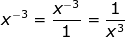1st write with a "top floor" and "bottom floor"

2nd change floors if the exponent is "unhappy"

•The exponent is unhappy in the denominator so
move to the numerator and it becomes positive.

Let's look at some more challenging examples

•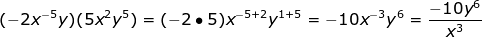•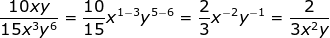•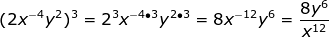Remember to work slowly and meticulously. You will need to memorize the rules for exponents. A shortened version:

Divide → Subtract exponents

Power to a power → Multiply exponents

Negative → Change "floors"

 Related Links: Math algebra Exponents Exponents and Powers The Laws of Exponents Integers as Exponents Evaluate Exponents Evaluate integers with exponents Rational (Fractional) Exponents Rational (Fractional) Exponents Zero Exponents

To link to this Positive and Negative Integer Exponents page, copy the following code to your site: